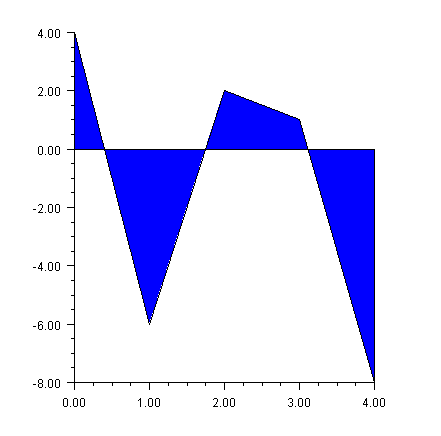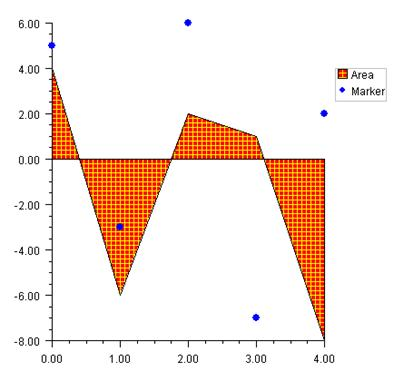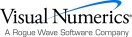## Area Plot

Area plots are similar to line plots, but with the area between the line and a refer­ence line filled in.  An area plot is created if the DATA_TYPE_FILL bit is on in the value of the DataType attribute.  The default reference line is y = 0. The location of the reference line can be changed from 0 by setting the Reference property. The Fill Area Attributes determine how the area is filled.

### Simple Area Plot

This example draws a simple area plot. The default value of the FillType attribute is FILL_TYPE_SOLID. The example sets the FillColor attribute to Color.Blue. So the area between the line y = 0 is solid blueusing Imsl.Chart2D;

using System.Drawing;

public class SampleArea : FrameChart {

public SampleArea() {

Chart chart = this.Chart;

AxisXY axis = new AxisXY(chart);

double[] y = new double[] {4, -6, 2, 1, -8};

Data data1 = new Data(axis, y);

data1.DataType = Data.DATA_TYPE_FILL;

data1.FillColor = Color.Blue;

}

public static void Main(string[] argv) {

System.Windows.Forms.Application.Run(new SampleArea());

}

}

### Painted Area Example

This example shows an area chart filled in with a painted texture. The texture is cre­ated by a static method FillPaint.Crosshatch.

A second data set is plotted as a set of markers.

The Legend node is painted in this example and has entries for both the filled area data set and the marker data set.using Imsl.Chart2D;

using System.Drawing;

public class SampleAreaPaint : FrameChart {

public SampleAreaPaint() {

Chart chart = this.Chart;

AxisXY axis = new AxisXY(chart);

chart.Legend.IsVisible = true;

double[] y = new double[] {4, -6, 2, 1, -8};

Data data1 = new Data(axis, y);

data1.SetTitle("Area");

data1.DataType = Data.DATA_TYPE_FILL;

data1.FillType = Data.FILL_TYPE_PAINT;

data1.SetFillPaint(FillPaint.Crosshatch(

10, 5, Color.Red, Color.Yellow));

double[] y2 = {5, -3, 6, -7, 2};

Data data2 = new Data(axis, y2);

data2.SetTitle("Marker");

data2.DataType = Data.DATA_TYPE_MARKER;

data2.MarkerColor = Color.Blue;

data2.MarkerType = Data.MARKER_TYPE_FILLED_CIRCLE;

}

public static void Main(string[] argv) {

System.Windows.Forms.Application.Run(new SampleAreaPaint());

}

}

### Attribute Reference

The attribute Reference defines the reference line. If its value is a, then the reference line is y = a. Its default value is 0.Visual Numerics - Developers of IMSL and PV-WAVEhttp://www.vni.com/PHONE: 713.784.3131FAX:713.781.9260